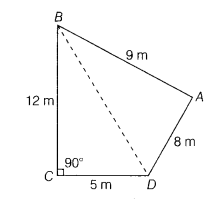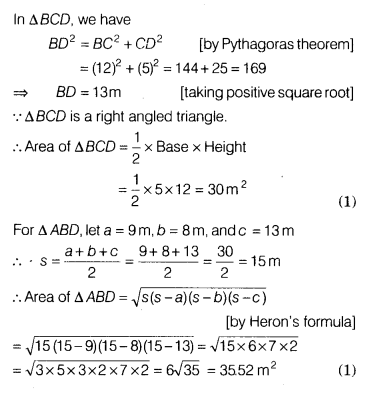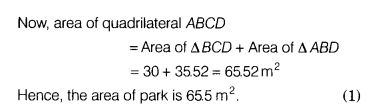# A park in the shape of quadrilateral ABCD has ∠C - 90°, AB = 9 m, BC = 12 m

A park in the shape of quadrilateral ABCD has ∠C - 90°, AB = 9 m, BC = 12 m,
CD =5m and AD =8m. How much area does it occupy?

Given, a quadrilateral ABCD in which AB = 9 m, BC = 12 m, CD = 5 m, AD = 8 m, and∠C = 90°.
Join the diagonal BD.